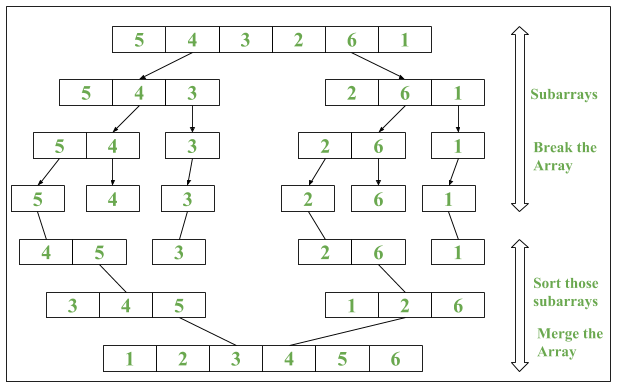# Java 8 | Arrays parallelSort() method with Examples

• Difficulty Level : Easy
• Last Updated : 11 Dec, 2018

Java 8 introduced a new method called as parallelSort() in java.util.Arrays Class. It uses Parallel Sorting of array elements

Algorithm of parallelSort()

```1. The array is divided into sub-arrays and that
sub-arrays is again divided into their sub-arrays,
until the minimum level of detail in a set of array.
2. Arrays are sorted individually by multiple thread.
3. The parallel sort uses Fork/Join Concept for sorting.
4. Sorted sub-arrays are then merged.```

Syntax :

1. For sorting data in ascending order :
```public static void parallelSort(Object obj[])
```
2. For sorting data in specified range in ascending order :
`public static void parallelSort(Object obj[], int from, int to)`

parallelSort() method uses concept of MultiThreading which makes the sorting faster as compared to normal sorting method.

ExampleBelow are the program that will illustrate the use of Arrays.parallelSort():

Program 1: To demonstrate use of Parallel Sort

 `// Java program to demonstrate ` `// Arrays.parallelSort() method ` ` `  `import` `java.util.Arrays; ` ` `  `public` `class` `ParallelSort { ` `    ``public` `static` `void` `main(String[] args) ` `    ``{ ` `        ``// Creating an array ` `        ``int` `numbers[] = { ``9``, ``8``, ``7``, ``6``, ``3``, ``1` `}; ` ` `  `        ``// Printing unsorted Array ` `        ``System.out.print(``"Unsorted Array: "``); ` `        ``// Iterating the Elements using stream ` `        ``Arrays.stream(numbers) ` `            ``.forEach(n -> System.out.print(n + ``" "``)); ` `        ``System.out.println(); ` ` `  `        ``// Using Arrays.parallelSort() ` `        ``Arrays.parallelSort(numbers); ` ` `  `        ``// Printing sorted Array ` `        ``System.out.print(``"Sorted Array: "``); ` `        ``// Iterating the Elements using stream ` `        ``Arrays.stream(numbers) ` `            ``.forEach(n -> System.out.print(n + ``" "``)); ` `    ``} ` `} `

Output:

```Unsorted Array: 9 8 7 6 3 1
Sorted Array: 1 3 6 7 8 9
```

Time Complexity is O(nlogn)

Program 2: To demonstrate use of Parallel Sort w.r.t. Series Sort (Normal Sort)

 `// Java program to demonstrate impact  ` `// of Parallel Sort vs Serial Sort  ` ` `  `import` `java.util.Arrays;  ` `import` `java.util.Random;  ` ` `  `public` `class` `ParallelSort {  ` `    ``public` `static` `void` `main(String[] args)  ` `    ``{  ` `        ``// Creating an array  ` `        ``int` `numbers[] = ``new` `int``[``100``];  ` ` `  `        ``// Iterating Loop till i = 1000  ` `        ``// with interval of 10  ` `        ``for` `(``int` `i = ``0``; i < ``1000``; i += ``10``) {  ` ` `  `            ``System.out.println(``"\nFor iteration number: "` `                            ``+ (i / ``10` `+ ``1``));  ` ` `  `            ``// Random Int Array Generation  ` `            ``Random rand = ``new` `Random();  ` ` `  `            ``for` `(``int` `j = ``0``; j < ``100``; j++) {  ` `                ``numbers[j] = rand.nextInt();  ` `            ``}  ` ` `  `            ``// Start and End Time of Arrays.sort()  ` `            ``long` `startTime = System.nanoTime();  ` ` `  `            ``// Performing Serial Sort  ` `            ``Arrays.sort(numbers);  ` ` `  `            ``long` `endTime = System.nanoTime();  ` ` `  `            ``// Printing result of Serial Sort  ` `            ``System.out.println(``"Start and End Time in Serial (in ns): "` `                            ``+ startTime + ``":"` `+ endTime);  ` `            ``System.out.println(``"Time taken by Serial Sort(in ns): "` `                            ``+ (endTime - startTime));  ` ` `  `            ``// Start and End Time of Arrays.parallelSort()  ` `            ``startTime = System.nanoTime();  ` ` `  `            ``// Performing Parallel Sort  ` `            ``Arrays.parallelSort(numbers);  ` ` `  `            ``endTime = System.nanoTime();  ` ` `  `            ``// Printing result of Parallel Sort  ` `            ``System.out.println(``"Start and End Time in parallel (in ns): "` `                            ``+ startTime + ``":"` `+ endTime);  ` `            ``System.out.println(``"Time taken by Parallel Sort(in ns): "` `                            ``+ (endTime - startTime));  ` `            ``System.out.println();  ` `        ``}  ` `    ``}  ` `}  `

Output:

```For iteration number: 1
Start and End Time in Serial (in ns): 3951000637977:3951000870361
Time taken by Serial Sort(in ns): 232384
Start and End Time in parallel (in ns): 3951000960823:3951000971044
Time taken by Parallel Sort(in ns): 10221

For iteration number: 2
Start and End Time in Serial (in ns): 3951001142284:3951001201757
Time taken by Serial Sort(in ns): 59473
Start and End Time in parallel (in ns): 3951001256643:3951001264039
Time taken by Parallel Sort(in ns): 7396
.
.
.
For iteration number: 99
Start and End Time in Serial (in ns): 3951050723541:3951050731520
Time taken by Serial Sort(in ns): 7979
Start and End Time in parallel (in ns): 3951050754238:3951050756130
Time taken by Parallel Sort(in ns): 1892

For iteration number: 100
Start and End Time in Serial (in ns): 3951050798392:3951050804741
Time taken by Serial Sort(in ns): 6349
Start and End Time in parallel (in ns): 3951050828544:3951050830582
Time taken by Parallel Sort(in ns): 2038
```

Note : Different time intervals will be printed But parallel sort will be done before normal sort.

Environment: 2.6 GHz Intel Core i7, java version 8

My Personal Notes arrow_drop_up
Recommended Articles
Page :